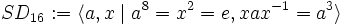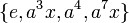# Semidihedral group:SD16

View a complete list of particular groups (this is a very huge list!)[SHOW MORE]

## Definition

The semidihedral group$SD_{16}$ (also denoted$QD_{16}$) is the semidihedral group (also called quasidihedral group) of order$16$. Specifically, it has the following presentation:$SD_{16} := \langle a,x \mid a^8 = x^2 = e, xax^{-1} = a^3 \rangle$.

The group can also be defined as the general semilinear group of degree one over the field of nine elements.

## Arithmetic functions

Want to compare and contrast arithmetic function values with other groups of the same order? Check out groups of order 16#Arithmetic functions
Function Value Similar groups Explanation for function value
underlying prime of p-group 2
order (number of elements, equivalently, cardinality or size of underlying set) 16 groups with same order
prime-base logarithm of order 4 groups with same prime-base logarithm of order
max-length of a group 4 max-length of a group equals prime-base logarithm of order for group of prime power order
chief length 4 chief length equals prime-base logarithm of order for group of prime power order
composition length 4 composition length equals prime-base logarithm of order for group of prime power order
exponent of a group 8 groups with same order and exponent of a group | groups with same exponent of a group cyclic subgroup of order 8.
prime-base logarithm of exponent 3 groups with same order and prime-base logarithm of exponent | groups with same prime-base logarithm of order and prime-base logarithm of exponent | groups with same prime-base logarithm of exponent
nilpotency class 3 groups with same order and nilpotency class | groups with same prime-base logarithm of order and nilpotency class | groups with same nilpotency class
derived length 2 groups with same order and derived length | groups with same prime-base logarithm of order and derived length | groups with same derived length the derived subgroup is contained in the cyclic subgroup and is hence abelian
Frattini length 3 groups with same order and Frattini length | groups with same prime-base logarithm of order and Frattini length | groups with same Frattini length
minimum size of generating set 2 groups with same order and minimum size of generating set | groups with same prime-base logarithm of order and minimum size of generating set | groups with same minimum size of generating set
subgroup rank of a group 2 groups with same order and subgroup rank of a group | groups with same prime-base logarithm of order and subgroup rank of a group | groups with same subgroup rank of a group All proper subgroups are cyclic, dihedral, semidihedral or Klein four-groups.
rank of a p-group 2 groups with same order and rank of a p-group | groups with same prime-base logarithm of order and rank of a p-group | groups with same rank of a p-group there exist Klein four-subgroups.
normal rank of a p-group 1 groups with same order and normal rank of a p-group | groups with same prime-base logarithm of order and normal rank of a p-group | groups with same normal rank of a p-group all abelian normal subgroups are cyclic.
characteristic rank of a p-group 1 groups with same order and characteristic rank of a p-group | groups with same prime-base logarithm of order and characteristic rank of a p-group | groups with same characteristic rank of a p-group All abelian characteristic subgroups are cyclic.

Note that the order can also be computed using the formula for the order of$\Gamma L(1,p^r)$ where$p = 3, r = 2$ and$q = p^r = 9$: The order of$\Gamma L(1,q)$ with$q = p^r$ is$r(q - 1)$, which becomes$2(9 - 1) = 2(8) = 16$.

### Arithmetic functions of a counting nature

Function Value Similar groups Explanation
number of conjugacy classes 7 groups with same order and number of conjugacy classes | groups with same prime-base logarithm of order and number of conjugacy classes | groups with same number of conjugacy classes As$SD_{2^n}, n = 4$:$2^{n-2} + 3 = 2^2 + 3 = 7$
As$\Gamma L(1,p^2), p = 3$:$(p^2 + 3p - 4)/2$ which becomes$(3^2 + 3 \cdot 3 - 4)/2 = 7$. See element structure of general semilinear group of degree one over a finite field.
See element structure of semidihedral group:SD16
number of equivalence classes under rational conjugacy 6 groups with same order and number of equivalence classes under rational conjugacy | groups with same prime-base logarithm of order and number of equivalence classes under rational conjugacy | groups with same number of equivalence classes under rational conjugacy
number of conjugacy classes of rational elements 5 groups with same order and number of conjugacy classes of rational elements | groups with same prime-base logarithm of order and number of conjugacy classes of rational elements | groups with same number of conjugacy classes of rational elements

## Group properties

### Important properties

Property Satisfied Explanation Comment
group of prime power order Yes
nilpotent group Yes prime power order implies nilpotent
supersolvable group Yes via nilpotent: finite nilpotent implies supersolvable
solvable group Yes via nilpotent: nilpotent implies solvable
abelian group No
metacyclic group Yes has a cyclic subgroup of order 8, with cyclic quotient group
metabelian group Yes follows from being metacyclic; also, from having class three.

### Other properties

Property Meaning Satisfied? Explanation Comment
group in which every normal subgroup is characteristic every normal subgroup of the group is a characteristic subgroup of it. Yes
maximal class group finite p-group that is non-abelian and whose nilpotency class is one less than its prime-base logarithm of order. Yes
UL-equivalent group nilpotent group whose upper central series and lower central series coincide. Yes follows from being a maximal class group
stem group center is contained in the derived subgroup. Yes
directly indecomposable group nontrivial and cannot be expressed as an internal direct product of proper nontrivial subgroups. Yes
centrally indecomposable group nontrivial and cannot be expressed as an internal central product of proper nontrivial subgroups. Yes
splitting-simple group nontrivial and cannot be expressed as an internal semidirect product of proper nontrivial subgroups. No it is an internal semidirect product of the normal subgroup$\langle a \rangle$ and the group$\langle x \rangle$.
finite group with periodic cohomology finite group in which every abelian subgroup is cyclic. For the finite p-group case, this is equivalent to the rank being one. No
Schur-trivial group the Schur multiplier is trivial. Yes
ambivalent group every element is conjugate to its inverse. No$a,a^{-1}$ are not conjugate.
ACIC-group every automorph-conjugate subgroup is characteristic No$\langle a^4,x \rangle$ is automorph-conjugate but not characteristic.
T-group every subnormal subgroup is normal No$\langle x \rangle$ is subnormal but not normal. Also, not a Dedekind group. In fact, for nilpotent groups, being a T-group is equivalent to being a Dedekind group.

## Subgroups

Further information: Subgroup structure of semidihedral group:SD16

Automorphism class of subgroups List of subgroups Isomorphism class Order of subgroups Index of subgroups Number of conjugacy classes (=1 iff automorph-conjugate subgroup) Size of each conjugacy class (=1 iff normal subgroup) Total number of subgroups (=1 iff characteristic subgroup) Isomorphism class of quotient (if subgroup is normal) Subnormal depth (if proper and normal, this equals 1) Nilpotency class
trivial subgroup$\{ e \}$ trivial group 1 16 1 1 1 semidihedral group:SD16 1 0
center of semidihedral group:SD16$\{ e, a^4 \}$ cyclic group:Z2 2 8 1 1 1 dihedral group:D8 1 1
non-normal order two subgroups of semidihedral group:SD16$\{ e, x \}, \{ e, a^2x \}, \{ e, a^4x \}, \{ e, a^6x\}$ cyclic group:Z2 2 8 1 4 4 -- 3 1
derived subgroup of semidihedral group:SD16$\{ e, a^2, a^4, a^6 \}$ cyclic group:Z4 4 4 1 1 1 Klein four-group 1 1
non-normal order four cyclic subgroups of semidihedral group:SD16$\{ e, ax, a^4, a^5x \}$,$\{ e, a^3x, a^4, a^7x \}$ cyclic group:Z4 4 4 1 2 2 -- 2 1
Klein four-subgroups of semidihedral group:SD16$\{ e, x, a^4, a^4x \}$,$\{ e, a^2x, a^4, a^6x \}$ Klein four-group 4 4 1 2 2 -- 2 1
Z8 in SD16$\langle a \rangle$ cyclic group:Z8 8 2 1 1 1 cyclic group:Z2 1 1
D8 in SD16$\langle a^2, x \rangle$ dihedral group:D8 8 2 1 1 1 cyclic group:Z2 1 2
Q8 in SD16$\langle a^2, ax \rangle$ quaternion group 8 2 1 1 1 cyclic group:Z2 1 2
whole group all elements semidihedral group:SD16 16 1 1 1 1 trivial group 0 3
Total (10 rows) -- -- -- -- 10 -- 15 -- -- --

## Subgroup-defining functions

Subgroup-defining function Subgroup type in list Page on subgroup embedding Isomorphism class Comment
Center (2) cyclic group:Z2
Commutator subgroup (4) cyclic group:Z4
Frattini subgroup (4) cyclic group:Z4
Socle (2) cyclic group:Z4
Join of abelian subgroups of maximum order (7) cyclic group:Z8
Join of abelian subgroups of maximum rank (8) dihedral group:D8
Join of elementary abelian subgroups of maximum order (8) dihedral group:D8

## GAP implementation

### Group ID

This finite group has order 16 and has ID 8 among the groups of order 16 in GAP's SmallGroup library. For context, there are 14 groups of order 16. It can thus be defined using GAP's SmallGroup function as:

SmallGroup(16,8)

For instance, we can use the following assignment in GAP to create the group and name it$G$:

gap> G := SmallGroup(16,8);

Conversely, to check whether a given group$G$ is in fact the group we want, we can use GAP's IdGroup function:

IdGroup(G) = [16,8]

or just do:

IdGroup(G)

to have GAP output the group ID, that we can then compare to what we want.

### Hall-Senior number

This group of prime power order has order 16 and has Hall-Senior number 13 among the groups of order 16. This information can be used to construct the group in GAP using the Gap3CatalogueGroup function as follows:

Gap3CatalogueGroup(16,13)

WARNING: There is some disagreement between the GAP 3 catalogue numbers and the Hall-Senior numbers for some abelian groups, but it does not affect this group.

For instance, we can use the following assignment in GAP to create the group and name it$G$:

gap> G := Gap3CatalogueGroup(16,13);

Conversely, to check whether a given group$G$ is in fact the group we want, we can use GAP's Gap3CatalogueIdGroup function:

Gap3CatalogueIdGroup(G) = [16,13]

or just do:

Gap3CatalogueIdGroup(G)

to have GAP output the group ID, that we can then compare to what we want.

### Description by presentation

The group can be defined using a presentation as follows:

gap> F := FreeGroup(2);;
gap> G := F/[F.1^8,F.2^2,F.2*F.1*F.2*F.1^(-3)];
<fp group on the generators [ f1, f2 ]>
gap> IdGroup(G);
[ 16, 8 ]

### Other descriptions

Description Functions used
SylowSubgroup(GL(2,3),2) SylowSubgroup, GL